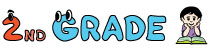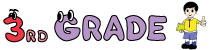1. Home>
2. Math>
3. Math Quiz>
4. Multiplication Quiz

# Multiplication Quiz - Online Multiplication Practice

Multiplication quizzes contain over 100 skills from 2nd grade through 4th grade. It contains online practices in writing multiplication sentences using models, times tables, multiplication facts, horizontal and vertical multiplication, drills, estimation and more.2nd grade multiplication practice contains identifying and completing multiplication sentences using real-life models, times tables up to 5, missing factors and more quizzes.Third grade multiplication practice contains understanding multiplication using equal groups, arrays and number lines, quizzes on multiplication times tables up to 12, repeated addition, multiplication properties and more.Fourth grade multiplication practice contains balancing equations, comparing products, in-out boxes, multiplying numbers with more digits, estimation quiz and more.